# Question Bank Solutions for SSC (English Medium) 6th Standard - Maharashtra State Board - Mathematics

Subjects
Topics
Subjects
Popular subjects
Topics
Mathematics
< prev  1 to 20 of 344  next >

Look at the figure alongside and name the following:

Collinear points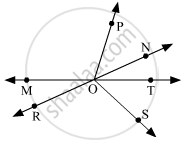[0.01] Basic Concepts in Geometry
Chapter: [0.01] Basic Concepts in Geometry
Concept: Basic Concept in Geometry

Look at the figure alongside and name the following:

Rays[0.01] Basic Concepts in Geometry
Chapter: [0.01] Basic Concepts in Geometry
Concept: Basic Concept in Geometry

Look at the figure alongside and name the following:

Line segments[0.01] Basic Concepts in Geometry
Chapter: [0.01] Basic Concepts in Geometry
Concept: Basic Concept in Geometry

Look at the figure alongside and name the following:

Lines[0.01] Basic Concepts in Geometry
Chapter: [0.01] Basic Concepts in Geometry
Concept: Basic Concept in Geometry

Write the different names of the line.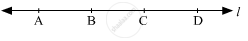[0.01] Basic Concepts in Geometry
Chapter: [0.01] Basic Concepts in Geometry
Concept: Basic Concept in Geometry

Different mathematical operations are given in the two rows below. Find out the number you get in each operation and make equations.

16 ÷ 2; 5 × 2; 9 + 4; 72 ÷ 3; 4 + 5; 8 × 3; 19 - 10; 10 - 2; 37 - 27; 6 + 7

[0.1] Equations
Chapter: [0.1] Equations
Concept: Concept of Equation

Match the Groups:

 Group A Group B (i)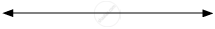(a) Ray (ii)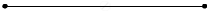(b) Plane (iii)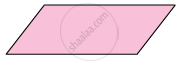(c) Line (iv)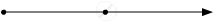(d) Line segment
[0.01] Basic Concepts in Geometry
Chapter: [0.01] Basic Concepts in Geometry
Concept: Basic Concept in Geometry

Observe the figure below. Name the parallel lines, the concurrent lines, and the points of concurrence in the figure.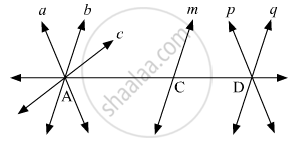[0.01] Basic Concepts in Geometry
Chapter: [0.01] Basic Concepts in Geometry
Concept: Basic Concept in Geometry

In the example below, find the proportion of the first number to the second.

24, 56

[0.11] Ratio and Proportion
Chapter: [0.11] Ratio and Proportion
Concept: Concept of Ratio

In the example below, find the proportion of the first number to the second.

63, 49

[0.11] Ratio and Proportion
Chapter: [0.11] Ratio and Proportion
Concept: Concept of Ratio

In the example below, find the proportion of the first number to the second.

52, 65

[0.11] Ratio and Proportion
Chapter: [0.11] Ratio and Proportion
Concept: Concept of Ratio

In the example below, find the proportion of the first number to the second.

84, 60

[0.11] Ratio and Proportion
Chapter: [0.11] Ratio and Proportion
Concept: Concept of Ratio

In the example below, find the proportion of the first number to the second.

35, 65

[0.11] Ratio and Proportion
Chapter: [0.11] Ratio and Proportion
Concept: Concept of Ratio

In the example below, find the proportion of the first number to the second.

121, 99

[0.11] Ratio and Proportion
Chapter: [0.11] Ratio and Proportion
Concept: Concept of Ratio

Find the ratio of the first quantity to the second.

[0.11] Ratio and Proportion
Chapter: [0.11] Ratio and Proportion
Concept: Concept of Ratio

Find the ratio of the first quantity to the second.

40 rupees, 120 rupees

[0.11] Ratio and Proportion
Chapter: [0.11] Ratio and Proportion
Concept: Concept of Ratio

Find the ratio of the first quantity to the second.

15 minutes, 1 hour

[0.11] Ratio and Proportion
Chapter: [0.11] Ratio and Proportion
Concept: Concept of Ratio

Find the ratio of the first quantity to the second.

30 litres, 24 litres

[0.11] Ratio and Proportion
Chapter: [0.11] Ratio and Proportion
Concept: Concept of Ratio

Find the ratio of the first quantity to the second.

99 kg, 44000 grams

[0.11] Ratio and Proportion
Chapter: [0.11] Ratio and Proportion
Concept: Concept of Ratio

Find the ratio of the first quantity to the second.

1 litre, 250 ml

[0.11] Ratio and Proportion
Chapter: [0.11] Ratio and Proportion
Concept: Concept of Ratio
< prev  1 to 20 of 344  next >